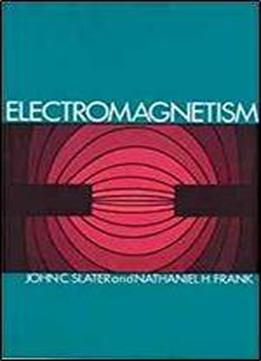Electromagnetism (dover Books On Physics) by John C. Slater / 2011 / English / DjVu

Clearly developed from first principles, this introductory study supplies basic material on electrostatics and magnetostatics, then concentrates on electromagnetic theory — the authors are both leading men in the field. The book ranges freely over many areas of electromagnetic theory with some concern for electrical engineering. It covers the field theory of electromagnetism, electrostatics and the equations and theorems of Gauss, Poisson, Laplace and Green, solutions of Laplace's equation, dielectrics, magnetic fields of linear and circular currents, electromagnetic induction and Maxwell's equations, electromagnetic waves, electron theory, wave guides and cavity resonators, spherical electromagnetic waves, Huygen's principle and Green's theorem, and Fresnel and Fraunhofer diffraction. Practice problems are supplied at chapter ends. Physicists and engineers will find this presentation particularly useful but mathematicians have also used the book not only as an introduction to electromagnetism, but also as a means to an increased knowledge of the aims and tools of theoretical physics. The only background required to follow the development is a knowledge of the calculus and differential equations. More advanced mathematics is developed in appendixes.

views: 387